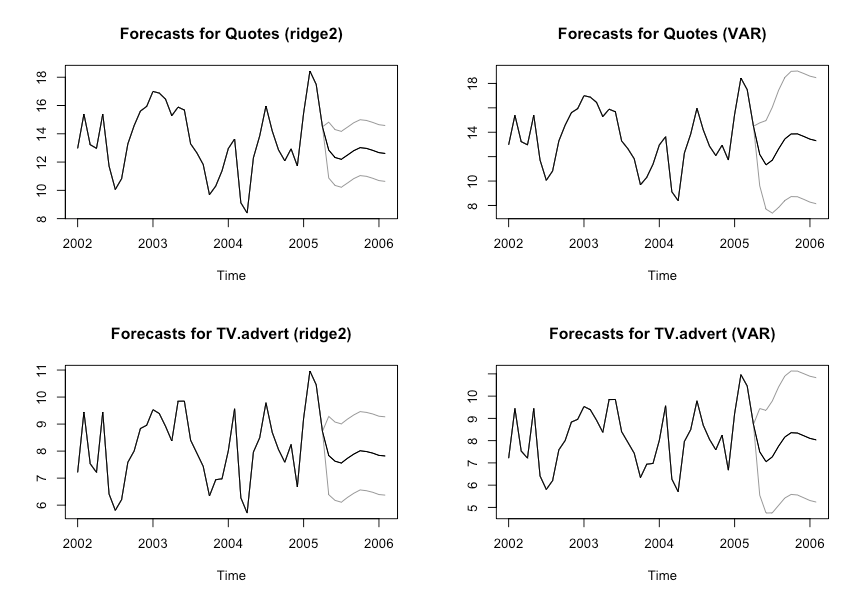Want to share your content on R-bloggers? click here if you have a blog, or here if you don't.

A few weeks ago, I introduced a Forecasting API that I deployed on Heroku. Under the hood, this API is built on top of `ahead` (and through Python packages rpy2 and Flask); an R package for univariate and multivariate time series forecasting. As of October 13th, 2021, 5 forecasting methods are implemented in `ahead`:

• `armagarchf`: univariate time series forecasting method using simulation of an ARMA(1, 1) – GARCH(1, 1)
• `dynrmf`: univariate time series forecasting method adapted from `forecast::nnetar` to support any Statistical/Machine learning model (such as Ridge Regression, Random Forest, Support Vector Machines, etc.)
• `eatf`: univariate time series forecasting method based on combinations of `forecast::ets`, `forecast::auto.arima`, and `forecast::thetaf`
• `ridge2f`: multivariate time series forecasting method, based on quasi-randomized networks and presented in this paper
• `varf`: multivariate time series forecasting method using Vector AutoRegressive model (VAR, mostly here for benchmarking purpose)

Here’s how to install the package:

• 1st method: from R-universe

In R console:

```  options(repos = c(
techtonique = 'https://techtonique.r-universe.dev',
CRAN = 'https://cloud.r-project.org'))

```
• 2nd method: from Github

In R console:

```  devtools::install_github("Techtonique/ahead")
```
• Or

```  remotes::install_github("Techtonique/ahead")
```

And here are the packages that will be used for this demo:

```library(ahead)
library(fpp)
library(datasets)
library(randomForest)
library(e1071)
```

# Univariate time series

In this section, we illustrate `dynrmf` for univariate time series forecasting, using Random Forest and SVMs. Do not hesitate to type `?dynrmf`, `?armagarchf` or `?eatf` in R console for more details and examples.

```par(mfrow=c(2, 2))

# Plotting forecasts
# With a Random Forest regressor, an horizon of 20,
# and a 95% prediction interval
plot(dynrmf(fdeaths, h=20, level=95, fit_func = randomForest::randomForest,
fit_params = list(ntree = 50), predict_func = predict))

# With a Support Vector Machine regressor, an horizon of 20,
# and a 95% prediction interval
plot(dynrmf(fdeaths, h=20, level=95, fit_func = e1071::svm,
fit_params = list(kernel = "linear"), predict_func = predict))

plot(dynrmf(Nile, h=20, level=95, fit_func = randomForest::randomForest,
fit_params = list(ntree = 50), predict_func = predict))

plot(dynrmf(Nile, h=20, level=95, fit_func = e1071::svm,
fit_params = list(kernel = "linear"), predict_func = predict))

```# Multivariate time series

In this section, we illustrate `ridge2f` and `varf` forecasting for multivariate time series. Do not hesitate to type `?ridge2f` or `?varf` in R console for more details on both functions.

```# Forecast using ridge2
# With 2 time series lags, an horizon of 10,
# and a 95% prediction interval
fit_obj_ridge2 <- ahead::ridge2f(fpp::insurance, lags = 2,
h = 10, level = 95)

# Forecast using VAR
fit_obj_VAR <- ahead::varf(fpp::insurance, lags = 2,
h = 10, level = 95)

# Plotting forecasts
# fpp::insurance contains 2 time series, Quotes and TV.advert
par(mfrow=c(2, 2))
plot(fit_obj_ridge2, "Quotes")
plot(fit_obj_VAR, "Quotes")